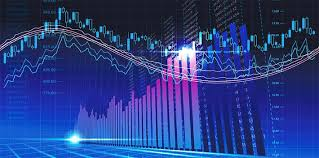### Ticker

6/recent/ticker-posts

# Guide to Fibonacci retracements in Forex trading

If you are a math enthusiast, you must have heard of the term ‘Fibonacci’. Now you must be wondering why we are suddenly talking about Fibonacci in relation to trading. Well, for your information, it is very important in the context of trading.

Have no idea what Fibonacci is? Don’t worry, we’ll soon enlighten you.

Fibonacci is a sequence in which you can find a number by adding the two previous numbers together. But here, 0 and 1 are the default numbers and the sequence generally starts with these two numbers. For your convenience, we will show you a Fibonacci sequence below.0,1,1,2,3,5,8,13,21,34…

In this sequence, the default numbers are zero and one.

So to find the next number, we add the two numbers before it.

0+1=1 which is the third term of the sequence

Again,

Adding the second and third term, we get,

1+2=3 which is the fourth term of the sequence

Adding the third and fourth term, we get

2+3=5 which is the fifth term of the sequence.

In this way, the sequence goes on to infinity.

The term Fibonacci was first coined by a famous Italian mathematician of the 12th century. In trading, Fibonacci is widely used not in the context of numbers but is derived from the mathematical relationship between numbers. It is mainly used as a frame for traders to look at. It neither helps to predict or contribute anything to the market, rather it allows traders to look at the trading decisions of other traders. This can be termed as a tool that influences traders’ decisions in trading.

This complex and time-consuming system can be used to predict the market up to 70% of the time and that is why it has become a necessary evil in trading. Feel free to visit the website and learn more about trading so that you can ease down the stress in the early stage.

## Fibonacci retracement

Fibonacci retracements indicate the horizontal lines where support and resistance zones are most likely to occur and each level is associated with a percentage. There are some commonly used percentages in this system which are 23.6%, 38.2%, 61.8% and 78.6%. Even though, a bit contradictable, 50% is also considered as one of the widely used percentage levels.

It is used to draw lines between two points in a price chart such as a high and a low creating the percentage levels between those two points.

The most fascinating part is that Fibonacci retracement is based on something called the golden ratio.

## The Golden ratio

Two indexes are considered as golden ratio when they proportionally increases its value in a sequential number. But the good thing is, you don’t have to depend on such complex calculation as you can draw the Fibonacci levels by using the critical highs and lows. But remember, the golden ratio is always an irrational number whose value is 1.6180339887…

We can say that all the terms in a Fibonacci sequence are approximately 1.618 times larger than its preceding number.

The inverse of the golden ratio number, 0,618 which is also used frequently in the trading sector.

## Now, how is Fibonacci retracement used in trading?

The horizontal lines drawn to indicate two levels in a price chart are used to identify possible price reversal points. Even though 50% per cent level is not based on a Fibonacci number, it is widely viewed by traders to predict potential price reversal points.

Traders use this system to know the percentage of an upward or downward trend that might bounce back to its initial position. As a result, traders try to make low-risk entries based on the predicted price level.

Fibonacci retracements are generally used alongside candlestick charts, trendlines, volume and momentum oscillators. It is widely used in fields like the stock market, commodity trading, crude oil trading and foreign exchange trading.

However, the only side effect of this complex system is that since it is completely based on predictions and average decisions made by other traders, the market speculation may not always be right which leads to an increase in potential risk levels.